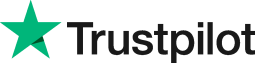Mode in Excel
• Home
• Courses
• Promotions
• Schedule
• Formats
• Our Clients

Forum home » Topics » Mode in Excel

# Mode in Excel

The Mode function in Excel returns the most frequent value in range of data.

This is different from the Average function which calculates the mean value or the Median function, which calculates the middle value.

Calculating the Mode in Excel may be more helpful when looking for a pattern or trend in the data. For example, finding the most common day of the week for staff absence or the most common answer to a question on a multiple choice questionnaire.

For many practical purposes it would be enough to calculate the average of a set of values, such as the average sales or average earnings.

For example suppose you ask 25 people for their shoe size and type these out in ascending order:

Shoe size
3
4
4
5
5
6
7
7
7
7
7
8
8
8
8
9
9
10
10
10
11
11
12
12
13

If you copy these values into range A1:A25 in Excel you can apply the functions: Count, Average, Mode and Median. Here are the results for this data:

Count = 25
Average = 8.04
Mode = 7
Median = 8

So for these 25 people the average size is 8.04 and the most frequent size is 7. The size of the person in the middle of the sorted data is 8.

# Related forum posts:

## Excel mean averages

What type of mean average are we using in the Excel.

how to i get an average for a column of figures

## Median values in a pivot table

Is there any way of getting median values into pivot tables?

 Welcome. Please choose your application (eg. Excel) and then post your question. Our Microsoft Qualified trainers will then respond within 24 hours (working days). Frequently Asked Questions What does 'Resolved' mean? Any suggestions, questions or comments? Please post in the Improve the forum thread.TrustScore 4.8 / 5       1238 reviews